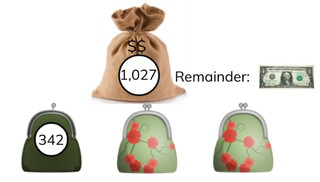Dividing numbers greater than 1,000 with remainder

# Dividing numbers greater than 1,000 with remainder

Students learn to divide numbers greater than 1,000 with remainder.8,000 schools use Gynzy92,000 teachers use Gynzy1,600,000 students use Gynzy

## General

The students learn to divide numbers greater than 1,000 with remainder.

## Standards

CCSS.Math.Content.5.NBT.B.5

## Learning objective

Students will be able to divide a number greater than 1,000 by a number less than 1,000, with remainder.

## Introduction

Have the students solve the division problems without remainder.

## Instruction

Explain that in division problems with a number greater than 1,000 with remainder you first see what number from the table of the divisor is the closest but less than the dividend. For the problem 1209 ÷ 40 you are looking for the largest number that you can take away. 3 × 40 = 120. So 30 × 40 = 1,200. Subtract the 1,200 from the 1,209. You now have 9 left over. You cannot divide 9 any more by 40. So 9 is your remainder. Discuss with the students the different methods of solving the problem 6531 ÷ 3. Then solve the other two problems and discuss the different methods in which these problems can be solved. Have the students solve the next three division problems on their own. Show the problem with the trains and the passengers and follow the steps to solve the problem. Emphasize that the remainder consists of 87 passengers, and these passengers also need to take a train. So to transport all of the passengers another train must ride that is not full. This is why your answer is not 31 R 87, but 32. Have the students solve the following division problems on their own. Point out that you have to carefully read the problem in order to determine whether they need to give the answer with or without remainder.

Check whether the students can divide a number greater than 1,000 with remainder by asking the following question:
- What steps do you follow to solve the problem 6,019 ÷ 20?

## Quiz

The students test their understanding of dividing numbers greater than 1,000 with remainder through ten exercises. For some of the exercises the students are given possible answers to a division problem to choose between, and for other exercises the students must provide the answer on their own. Some of the exercises are story problems.

## Closing

Discuss once again the importance of being able to divide a number greater than 1,000 with remainder. As a closing activity you can have the students solve the problem in pairs. First have them calculate the problem individually and then check their answers with their partner. Did they use the same steps? Could they have solved the problem using fewer steps?

## Teaching tips

Have students that have difficulty with dividing numbers greater than 1,000 with remainder first practice with the division tables and dividing numbers greater than 1,000 without remainder.

### The online teaching platform for interactive whiteboards and displays in schools

• Save time building lessons

• Manage the classroom more efficiently

• Increase student engagement